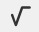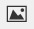PSABM6WB - Student Facing Task---Acc7.5 Lesson 10 Solutions to Linear Equations (8.EE.B, 8.EE.C)

There are many possible rectangles whose perimeter is 50 units. Give three possibilities for length and width of rectangles that give a perimeter of 50 units.

Part A)

At the corner produce market, apples cost \$1 each and oranges cost \$2 each.

Find the cost of:

6 apples and 3 oranges

Type your answer below as a number (example: 5, 3.1, 4 1/2, or 3/2):
Part B)

Find the cost of:

4 apples and 4 oranges

Type your answer below as a number (example: 5, 3.1, 4 1/2, or 3/2):
Part C)

Find the cost of:

5 apples and 4 oranges

Type your answer below as a number (example: 5, 3.1, 4 1/2, or 3/2):
Part D)

Find the cost of:

8 apples and 2 oranges

Type your answer below as a number (example: 5, 3.1, 4 1/2, or 3/2):
Part E)

Noah has \$10 to spend at the produce market. Can he buy 7 apples and 2 oranges?

Select one:
Part F)

Part G)

What combinations of apples and oranges can Noah buy if he spends all of his \$10?

Part H)

Use two variables to write an equation that represents \$10-combinations of apples and oranges. Be sure to say what each variable means.

Write the equation using the "WIRIS editor" buttonPart I)

What are 3 combinations of apples and oranges that make your equation true?

Part J)

What are three combinations of apples and oranges that make it false?

Part A)

Graph the equation you wrote relating the number of apples and the number of oranges.

Draw your graph on paper, take a picture, and upload it using the upload iconIf you do not have the ability to upload an image of your work, type "Graph is on paper."

Part B)

What is the slope of the graph? What is the meaning of the slope in terms of the context?

Part C)

Suppose Noah has \$20 to spend. Graph the equation describing this situation.

Draw your graph on paper, take a picture, and upload it using the upload iconIf you do not have the ability to upload an image of your work, type "Graph is on paper."

Part D)

What do you notice about the relationship between this graph and the earlier one?

Part A)

You have two numbers. If you double the first number and add it to the second number, the sum is 10.

Let x represent the first number and let y represent the second number. Write an equation showing the relationship between x, y, and 10.

Write the equation using the "WIRIS editor" buttonPart B)

Draw and label a set of x- and y-axes. Plot at least five points on this coordinate plane that make the statement and your equation true. What do you notice about the points you have plotted?

Draw your graph on paper, take a picture, and upload it using the image upload iconIf you do not have the ability to upload an image of your work, type "Graph is on paper."

Part C)

List ten points that do not make the statement true. Using a different color, plot each point in the same coordinate plane. What do you notice about these points compared to your first set of points?

Draw your graph on paper, take a picture, and upload it using the image upload iconIf you do not have the ability to upload an image of your work, type "Graph is on paper."